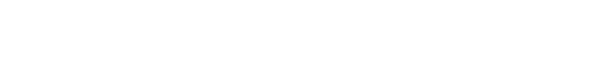# JavaScript/TypeScript currying arrow function

This week I saw an arrow function which has an arrow function. I didn’t know this but it seems to be called curried function or currying. It looks like the following.

const arrow = (x: number) => (y: number) => {
console.log(Arrow${x}-${y})
};

It is of course more complicated because it is production code but I couldn’t understand it at first look. After I asked my colleague I could finally understand. This is not an often-used technique but let’s learn how it works.

## Basic arrow function

Let’s look at the very basic arrow function first. The arrow function is the same as the lambda function in other languages. It is a function that doesn’t have a name. It looks like this.

const arrow = () => { console.log("Arrow1") };
arrow();
// Arrow1

If the function has one call we can omit {}. If the call returns a value caller can get the value without return keyword in this case.

const arrow = () => "Arrow2";
const result = arrow();
console.log(result);
// Arrow2

But when using {} we should add return keyword. In the following case, the arrow function returns nothing. In the result, the result variable becomes undefined.

const arrow = () => { "Arrow2-2" };
const result = arrow();
console.log(result - ${result}); // result - undefined Add return keyword if the function needs to return. const arrow = () => { return "Arrow2-3" }; const result = arrow(); console.log(result -${result});
// result - Arrow2-3

Next is with argument(s). Simply add arguments if arguments are necessary.

const arrow = (x: number) => { console.log(Arrow${x}) }; arrow(3); // Arrow3 Sponsored links ## Multi arrow function From here, it’s getting more difficult. Let’s look at this multi arrow function. const arrow = (x: number) => (y: number) => { console.log(Arrow${x}-${y}) }; const next = arrow(4); next(1); // Arrow4-1 As I wrote the result in the code section, it shows Arrow4-1. As I explained above, arrow functions return value if it has no curly brackets {} there. If we write it simpler with curly brackets {} it looks like this below.  const arrow = (x: number) => { const next = (y: number) => { console.log(Arrow${x}-${y}) }; next(1); } arrow(5); // Arrow5-1 This is easier to understand. Then, how does it look like if we want to return the function? const arrow = (x: number) => { const next = (y: number) => { console.log(Arrow${x}-${y}) }; return next; }; const result = arrow(6); result(1); // Arrow6-1 arrow function returns next function which requires y argument. So we need to pass number to result function. result function calls next function defined in arrow function. Then, it shows the console output. Let’s see the first function again. I hope you can understand what’s going on now. const arrow = (x: number) => (y: number) => { console.log(Arrow${x}-${y}) }; const next = arrow(4); next(1); // Arrow4-1 ## When to use currying arrow function We understand what it does but when can we use this technique? For example, this is useful if we want to define a setup function with a specified value in advance. Once the setup function is prepared we can trigger it whenever we want to do it. For example, when a class receives an event. Let’s see the actual example code. Following code is still a simple example but it is more difficult than the previous one. type Greeting = { timeout: number, name: string }; const createTimeouts = (greetings: Greeting[]) => (message: string) => { const setTimer = (greeting: Greeting) => { setTimeout(() => { console.log(${greeting.timeout} - ${message}${greeting.name});
}, greeting.timeout);
}
return greetings.map(value => setTimer(value));
};
const args: Greeting[] = [
{ timeout: 1000, name: "Foo" },
{ timeout: 3000, name: "Yuto" },
{ timeout: 5000, name: "Hoo" },
];
const setMessageTimer = createTimeouts(args);
setMessageTimer("Hello");
// 1000 - Hello Foo
// 3000 - Hello Yuto
// 5000 - Hello Hoo

It calls setTimout function 3 times at different times. The time is defined before it is actually called. When setMessageTimer function is called the 3 timers start.

We can also add as many arrow functions as we want.

const arrow =
(x: number) => (y: number) =>
(z: number) => (zz: number) => {
console.log(Arrow${x}-${y}-${z}-${zz})
};
arrow(7)(8)(9)(10);
// Arrow7-8-9-10

## Passing arrow function or function

Some functions require a callback. For those functions, we can pass an arrow function which we learned above. But we should know how it works because it may unintentionally behave when using this keyword.

Let’s see the following example.

 class Foo {
private foo = 55;
public doSomething(): void {
console.log(In Foo: ${this?.foo}); } } class MyCallback { private foo = 22; public do(callback: () => void) { console.log(In MyCallback:${this.foo});
callback();
}
}

do function requires a callback function. It works as expected if an arrow function is specified there.

const foo = new Foo();
const myCallback = new MyCallback();
myCallback.do(() => { foo.doSomething() });
// In MyCallback: 22
// In Foo: 55
myCallback.do(() => foo.doSomething());
// In MyCallback: 22
// In Foo: 55

But if we pass function itself it doesn’t work.

myCallback.do(foo.doSomething);
// In MyCallback: 22
// In Foo: undefined

This is because it passes a reference to foo.doSomething which means that it becomes just a function in the do function which doesn’t have this.

I thought this contents vary depending on how its function is called. In this case, I thought this.foo in Foo class showed 55. However, when I tried to build the following code build failed with this error 'this' implicitly has type 'any' because it does not have a type annotation..

function abc(){
console.log(this)
}

Anyway, we should always use the arrow function to pass a callback in order to prevent this unintentional behavior.

JavaScript/TypeScript